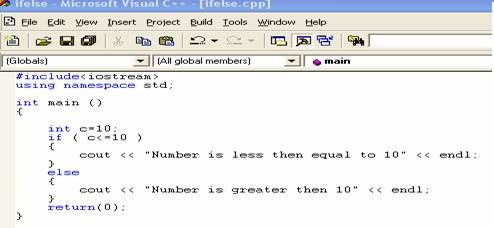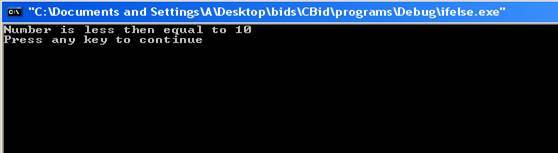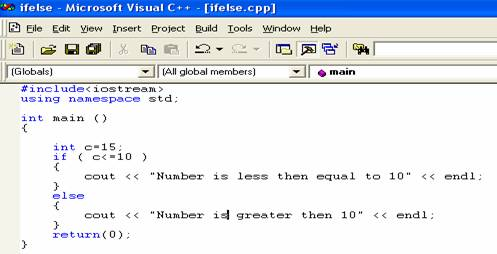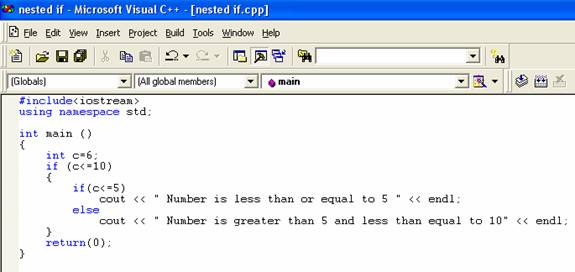If Else

Before reading this tutorial, you should have knowledge of math operators.

The if else statement is a type of control structure. A control structure is a instruction, statement or group of statements which determines the sequence of execution of other statements. The basic operation of if else statement is that a statement or group of statements is executed under if, if the value of expression is true and if the expression is false, statements under else are evaluated. In C++ language, zero is false everything else is true. Statement associated either with if or else are executed not both group of statements are executed. The else clause is optional. The general form of if else statement is:-

if (expression)

{

statement;

}

else

{

statement;

}

Or

if (expression)

{

statement;

}

The curly braces mark the beginning and the end statements under if and else.

Here is a program which illustrates the functioning of if else statement.The result of the program is:-The statement

if (c>=10)

checks whether the value of variable c is less than or equal to 10. The expression evaluates to be true and as a result statement

cout << “Number is less then equal to 10”

is executed. The statement

cout << “Number is greater then 10”

under else is not executed as value of the variable c is not greater than 10.

The position of semicolon is after the first statement following if and the expression. There is no semicolon after the expression in parentheses as if and expression are bounded to the statement or group of statements following the expression.  Here is the program which illustrates the working of statements under else.The result of the program is:-The statement

if (c>=10)

now evaluates to be false because the value of variable c is 15.  The statement under if is not executed. The statement

cout << “Number is greater then 10”

under else is executed as the expression evaluates to be false.

Nested ifs

The statement that is executed either after if or else can be itself be an if statement.  This arrangement is called nested if. In the nested if, the else statement is always associated with the nearest if statement within the same block. Here is a program which illustrates the working of nested ifs.The result of the program is: -The value of the variable c is 6. The statement

if (c<=10)

evaluates to be true. Then the statement

if (c<=5)

evaluates to be false and hence the statement

cout << “Number is greater than 5 and less than equal to 10”

under else in executed. It shows that else statement is associated with inner if statement.  Here is a program which makes things more clear.The result of the program is: -Now the value of the variable c is 12. The statement

if (c<=10)

evaluates to be false and hence the statement under else is executed. The statement

cout << “Number is greater than 10”

is executed as value of c is greater than 10. Now the else statement is associated with the outer if as else statement is outside the inner block.

After an introduction of if/else statements, let us move on to discuss switch statements.

Go to the previous lesson or proceed to the next lesson